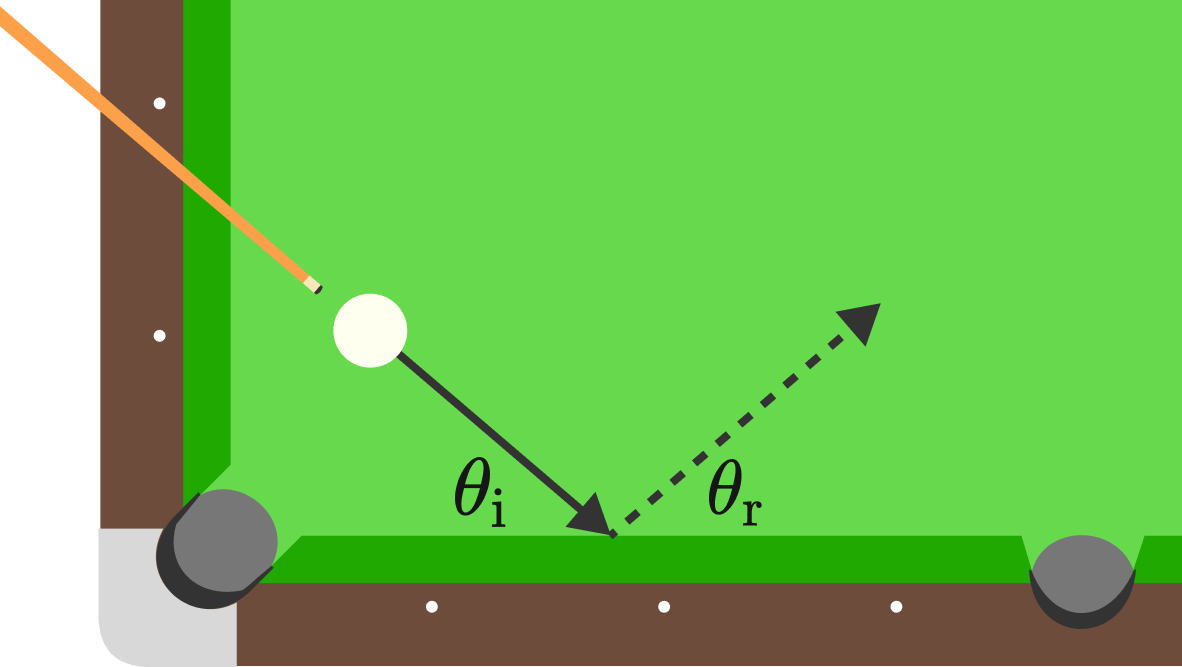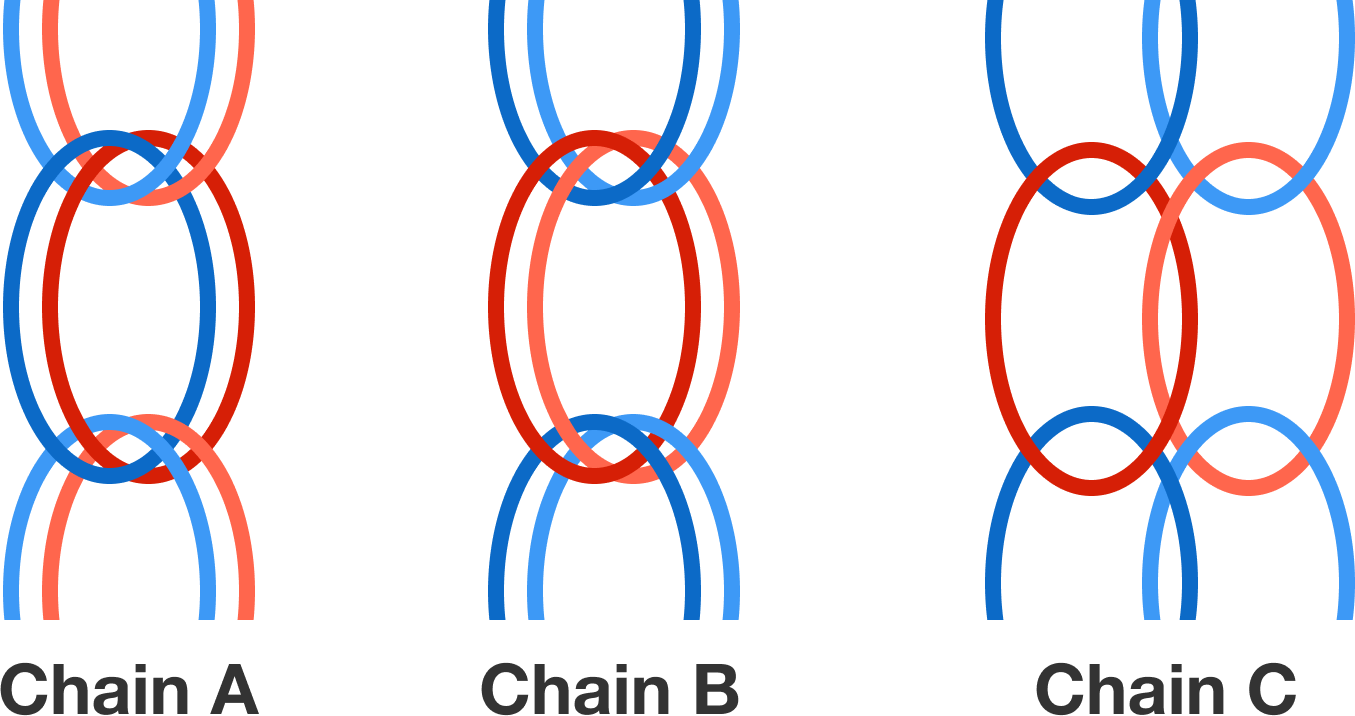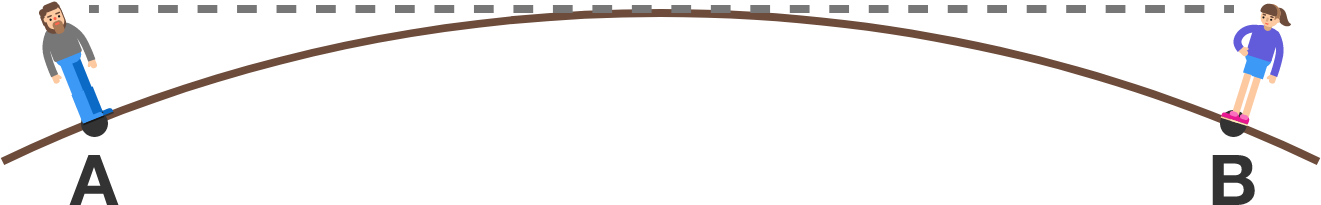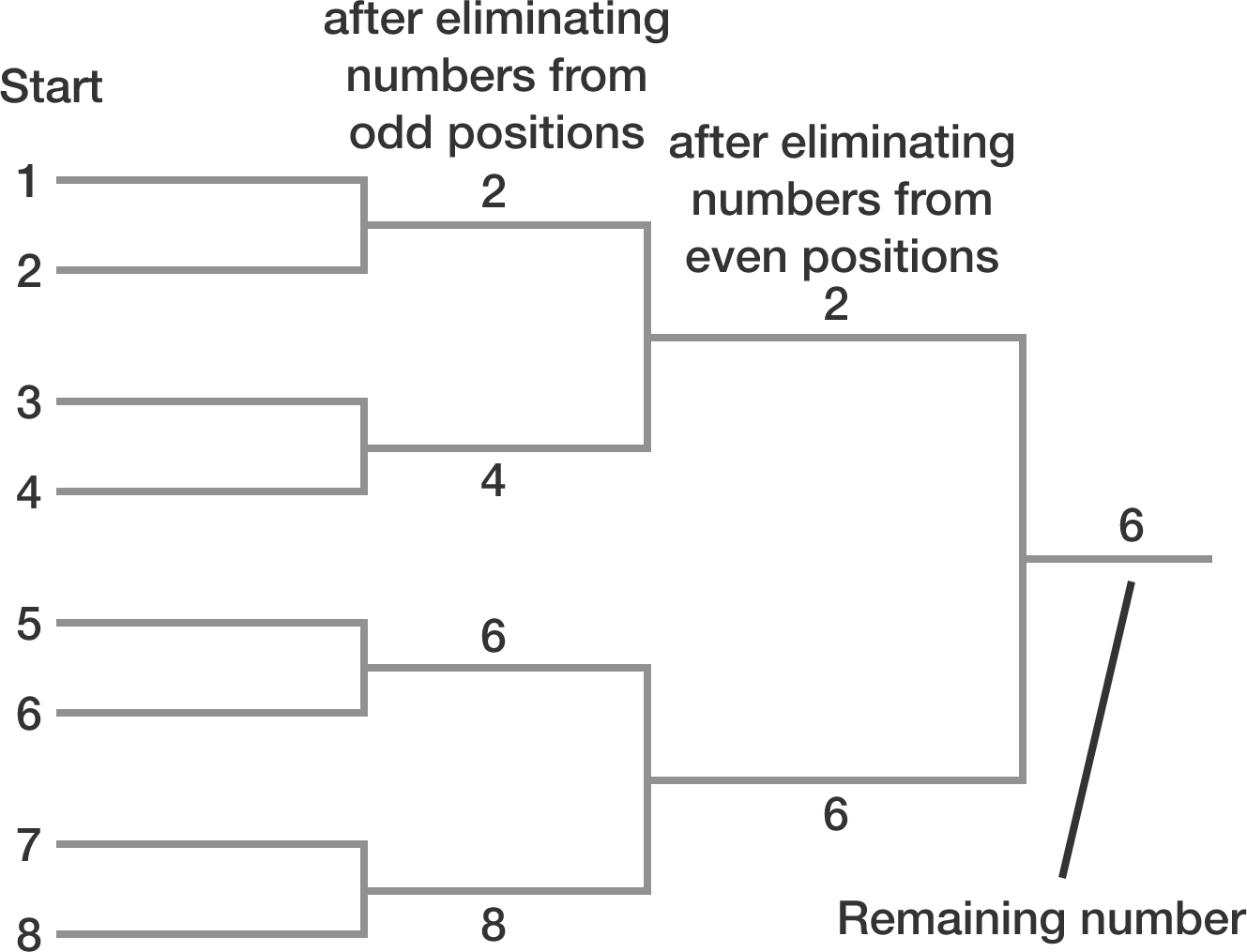# Problems of the Week

Contribute a problem

# 2018-01-22 Intermediate

Suppose we aim the cue ball at the cushion with some angle of incidence $\theta_\textrm{i} \neq 90^\circ.$ How will $\theta_\textrm{i}$ compare to $\theta_\textrm{r},$ the angle of reflection?

Naively it may seem that we can apply the law of reflection (that the angle of incidence is equal to the angle of reflection), but in practice we can't since some energy is lost upon colliding with the wall.Details and Assumptions:

• For simplicity, take the cue ball to be a point particle that has no sliding friction with the wall.
• The ball doesn't curve or jump on its way to the wall.

Fill in the blank:

$a$ and $b$ are positive integers such that $\hspace{20cm}$

• $a^b$ has exactly 5 positive divisors;
• $b^a$ has exactly 7 positive divisors;
• $a\times b$ has exactly $\text{\_\_\_\_\_\_\_}$ positive divisors.

Amy makes bracelets out of colorful rubber bands. Playing around with the rubber, she notices that the red rubber bands are much stiffer than the blue ones. To get uniformly elastic bracelets, she tries to combine red and blue bands in equal parts. As a first step, she makes three different types of chains from the rubber bands outlined in the figure. However, while trying out the chains, Amy is surprised to find that one chain is much stiffer than the other two. Which rubber chain is it?Hint: For small displacements $x$, a rubber band obeys Hook's law $f = - k x$ with reversing force $f$ and the force constant $k$. Figure out which force constant $k$ results when two different rubber bands with force constants $k_1$ and $k_2$ are linked in parallel or in series. (The chaining of rubber bands resembles the parallel and series connection in an electrical circuit.)

In 1838, Samuel Birley Rowbotham conducted observations leading him to conclude the earth was flat. His idea was the following: If light goes straight and I'm standing at $A$ with my friend, there must be a point $B$ where I can't see him.

Assuming light does not bend because of atmospheric refraction, which of these is closest to the minimum arc length of $\stackrel\frown{AB}$ that would prevent Samuel from seeing his friend? Assume they are both $1.8$ meters tall and the radius of the earth is $6,371,009\text{ m}.$Note: Though the earth is (of course) not flat, Samuel was able to see his friend at a farther distance than he was supposed to. This is due to atmospheric refraction, i.e. the non-homogeneous density of air creating a slight deviation of light. Without knowing this effect, a newspaper editor performed in 1896 the same experiment and concluded the earth was concavely curved.

The integers 1 through 12345 (inclusive) are written in a row in ascending order. You and your friend take turns removing numbers according to the following rules:

• Step 1: You remove numbers from all odd positions (i.e. 1, 3, 5, ..., 12345).
• Step 2: Your friend removes numbers from all even positions (i.e. 4, 8, ... because 2 is now in position #1 and 6 is in position #3, and so on).
• Step 3: If there is more than one number remaining on the list, repeat the steps above.What is the last number remaining after completing these steps?


Note:
The diagram to the right illustrates the case for a list of numbers 1 to 8.

×